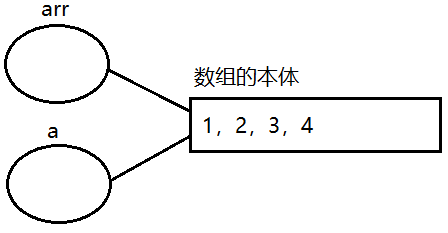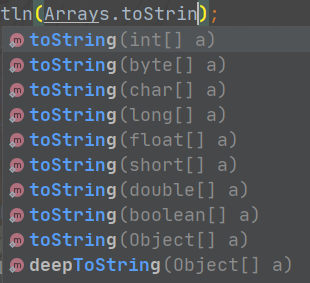• js数组传参
2021-11-24 14:25:11
var rows = grid.getSelectedRows();
var ids = new Array();
for(var j = 0; j < rows.length; j++) {
ids.push(rows[j].id);
}

传参：

data : {
"ids" : JSON.stringify(ids)
},

java接收“：

List<String> idList = JSONObject.parseArray(ids, String.class);开发语言
更多相关内容
• Java调用C语言动态库（JNA方式）：回调函数、结构体数组传参、结构体数组返回-附件资源
• 本文会介绍二维数组常见的三种传参方式，以整形数组为例。 1.int arr[行数][列数] int arr = { 1,2,3,4,0,1,2,3 }; 例如将上面的二维数组传入下方的函数 函数形参可以如下设置 void Print(int arr, ...

# 1.int arr[行数][列数]

int arr = { 1,2,3,4,0,1,2,3 };
例如将上面的二维数组传入下方的函数 函数形参可以如下设置
void Print(int arr, int m, int n)
{
int i = 0,j=0;
for (i = 0; i < m; i++)
{
for (j = 0; j < n; j++)
{
printf("%d ", arr[i][j]);
}
printf("\n");
}
}
实参传递方式如下
Print(arr, 2, 4);

# 3.以数组指针的方式传参

第三种方式也是本文着重介绍的方式

首先 介绍数组指针的定义方式

## 类型名 （*变量名）[一行的元素个数]

例如 int (*p)  表示p可以指向一个一行有五个元素的整形数组
注意：这里的"()"不能省略 否则上面的定义就成了一个指针数组了
对这里"()"的解释：首先清楚 我们要定义的是一个指针 而"[]"的优先级更高 因此我们要加"()"使得"*"优先与P结合 

了解了数组指针的定义方法，我们可以设计函数的形参了

int arr = { 1,2,3,4,0,1,2,3 };
int(*p) = arr;
将上面定义的数组传入下方函数，形参可设计如下
void Print(int(*p), int m, int n)
{
int i = 0,j=0;
for (i = 0; i < m; i++)
{
for (j = 0; j < n; j++)
{
printf("%d ", (*(p + i))[j]);
}
printf("\n");
}
}
实参传递方式：Print(p, 2, 4);
注意：对printf("%d ", (*(p + i))[j])的理解,p指向了二维数组的第一行，p+i 指向了二维数组第i行。解引用后我们就拿到了p+i行，即*(p+i)是p+i行的首元素地址。有了首元素地址 ，我们则可以按照 "(首元素地址)[下标]"的格式访问数组元素。 如果你理解了，就能理解另一种写法 前面提到*(p+i)是第i行首元素的地址
那么 *(p+i)+j 不就是第i行j列元素的地址  再解引用我们便能拿到地址中的内容 这便是另一种写法 *(*(p+i)+j)  

代码段里的东西很重要

展开全文c语言
• 数组的定义、遍历数组、引用 数组转字符串

# 1 数组的基本概念

## 1.1 数组的定义

数组，本质上就是，批量的创建一组相同类型的变量。
如果代码比较简单，只需要int a、int b两个数据就可以了，如果代码十分复杂需要创建一千个变量，我们可不能一个一个的 int 创建出来，这时候就需要 “ 规模制造 ”，用数组批量的一下子创建出来。注意，批量制造的一定得是***相同类型***。

## 1.2 数组的创建

三种数组的创建方式：

//1)数组的元素通过{}来指定
int[] arr1 = new int[]{1,2,3,4};
//2）数组的元素通过{}来指定
int[] arr2 = {1,2,3,4};         //一般写这样
//3）此时数组中的每个元素都被初始化成了0
//   这种写法主要用于指定长度
int[] arr3 = new int;

我们会发现，在C语言中，数组是int arr={1,2,3,4}; 这样写的。在Java中，int 和 [ ] 写在一起，它们原则上来说，应该是一个整体，共同构成了变量的类型。
在C语言中，int 和int 是两种不同的类型，也就不能相互赋值，但是在Java中就没有这个问题，不管数组长度如何，是一个类型，就可以相 互赋值。
注意 一种错误写法：
中括号中数和大括号不能同时存在，以此避免数和大括号中的个数不相同。

int arr4 = new int{1,2,3,4};     //编译出错

## 1.3 数组的使用

### 1.3.1 获取数组元素

和C语言类似，也是通过下标的方式来获取元素，不要忘记，大部分语言下标都是从 0 来时计算的，最后一个元素下标为length-1。使用下标访问的时候，不能超出有效范围，如果超出有效范围，在Java中会抛出一个数组下标越界异常

### 1.3.2 获取数组长度

数组一旦创建好了之后，长度（length）就固定了，无法动态进行修改。通过arr1.length 来获取数组的长度。

System.out.println(arr1.length);

注意：

1. 使用 arr.length 能够获取到数组的长度， 这个操作为成员访问操作符. 后面在面向对象中会经常用到。
2. 使用 [ ] 按下标取数组元素。 需要注意，下标从 0 开始计数。
3. 使用 [ ] 操作既能读取数据，也能修改数据。
4. 下标访问操作不能超出有效范围 [0, length - 1] ，如果超出有效范围，会出现下标越界异常。

### 1.3.3 数组的遍历

第一种方法：

int[] arr = {1,2,3,4};
for (int i = 0; i < arr.length ; i++) {
System.out.println(arr[i]);
}

第二种方法，foreach方法，更简洁，但注意不能通过x 对数组进行修改：

for (int x : arr){
System.out.println(x);
}

# 2 数组作为方法的参数

## 2.1 基本用法

(1) 数组赋值：

int[] arr = {1,2,3,4};
int[] a = arr;(2) 数组传参：
数组传参相当于 “ 赋值 ” 。赋值的时候，不是把原来的数组拷贝了一份形成新的数组，而是给原来的数组又起了个别名。数组是引用类型之一。
区分：内置类型在进行 = 的时候，不是起别名，而是创建了一个新的变量。

int[] arr = {1,2,3,4};
printArray(arr);
public static void printArray(int[] arr) {
for (int i = 0; i <arr.length ; i++) {
System.out.println(arr[i]);
}

通过别名修改数组，再从别的名字 “ 看 ” 都能看到

int[] arr = {1,2,3,4};
int[] a = arr;
transform(a);
printArray(arr);
public static void printArray(int[] arr) {
for (int i = 0; i <arr.length ; i++) {
System.out.println(arr[i]);
}
}
public static void transform(int[] a){
a = 100;
}

(3) 写一个方法，把数组中的每个元素都 * 2

public static int[] transform2(int[] a){
for (int i = 0; i <a.length ; i++) {
a[i] = a[i] * 2;
}
return a;
}

这样写，输出的结果就是乘二的数组，但是因为对a 直接进行操作，也就是通过别名对数组本体进行了操作改变，就无法保留原数组了。怎么样保留原数组呢，我们可以再创建一个数组。
为了保留了原来的数组，new 了一个新的数组，然后对新数组进行乘二的操作，再返回这个新数组，就可以既保留原数组又得到一个乘二的新数组。

public static int[] transform2(int[] a){
int[] result = new int[a.length];
for (int i = 0; i <a.length ; i++) {
result[i] = a[i] * 2;
}
return result;
}

要注意，这个逻辑在C语言中是会出现问题的，在C 中result 在函数执行完之后会被释放，result 会变成野指针，再对它进行操作就有问题了，但是，在Java 中可以。这是因为它们的生命周期不一样，即变量 ” 什么时候生成 什么时候释放 “ 的问题。在Java 中new 出来的对象的生命周期，是根据垃圾回收器自动判定的。

# 3 数组练习

## 3.1 数组转字符串

需求：查看数组的内容，希望可以更方便的观察，就可以把数组的内容按照一定的格式组织成一个字符串。
(1)我们可以写一个方法，将数组转换成String ，注意这中间的加号 “ + “ 是String字符串和数字，因此它执行的是字符串的拼接而不是数字相加的运算。

public static String arrayToString(int[] arr){
String result = "[";
for (int i = 0; i < arr.length; i++) {
result += arr[i];
if(i != arr.length-1){
result +=",";
}
}
result += "]";
return result;
}

(2) 标准库中其实有这样的方法我们可以直接使用，在Arrays中有这种方法，而且它重载了很多种类型。## 3.2 数组拷贝

(1) 我们可以写一个方法进行拷贝

public static int[] copyOf(int[] arr) {
int[] result = new int[arr.length];
for (int i = 0; i <arr.length ; i++) {
result[i] = arr[i];
}
return result;
}

(2) 同样也可以用标准库中的方法进行拷贝，我们可以看到，标准库的拷贝有两个参数，一个是数组名，一个是长度，如果新数组比原数组长度短，则相当于截断；如果新数组比原数组长度长，则长出来的部分自动用0 填充。

int[] result = Arrays.copyOf(arr,arr.length);

//拷贝某一部分
int[] result = Arrays.copyOfRange(arr, 2, 4);
System.out.println("result: " + Arrays.toString(result));

## 3.3 找数组中的最大元素

通过将一个位置固定当作 ” 擂台 “，然后其他的数来 ” 打擂台 “ 的方式进行比较，就是先固定一个位置，然后遍历其他的数与之比较大小，比它大就上去替代它，别的数再来比较，比擂台上的大就将擂台上的数 ” 打下擂台 “，大的数再站在擂台上，留到最后的就是最大的数。

public static int max(int[] arr) {
int result = arr;
for (int i = 0; i <arr.length ; i++) {
if(arr[i] > result){
result = arr[i];
arr[i] = result;
}
}
return result;
}

## 3.4 求平均值

即，将数组中的数全部加起来，然后处以数组的长度，来求得平均值，要注意平均值最后的返回值应该用double 类型，因为它不一定是整数。

public static double avg(int[] arr) {
int sum = 0;
for (int i = 0; i <arr.length ; i++) {
sum += i;
}
return  (double) sum/ arr.length;
}

## 3.5 查找数组中的指定元素

给定值，查找下标，就是找到指定元素的位置。

public static int search(int[] arr, int toSearch) {
int index = 0;
for (index = 0; index <arr.length ; index++) {
if(toSearch == arr[index]){
return index;
}
}
System.out.println("找不到该数。");
return -1;
}

## 3.6 查找数组中指定元素(二分查找)

针对有序数组, 可以使用更高效的二分查找，即，先找到这组有序数组的中间值，然后比较要找的元素和中间值，如果比中间值小，则把中间后面的 ” 砍掉 “，再在前半段找中间值，判断要找元素与中间值的大小，重复上述操作直至找到制定元素；如果比中间值大，则把中间值前面的 ” 砍掉 “，在前半段找中间值，判断要找元素与中间值的大小，重复上述操作直至找到制定元素。
详细可见 《Java实现二分查找 (详细) / 二分查找与普通查找效率》

public static int binarySearch(int[] a, int toSearch) {
int left = 0;
int right = a.length-1;
while (left <= right ) {
int mid = left + right / 2;
if( toSearch > a[mid] ){
//在后半段查找
left = mid + 1;
}else if( toSearch < a[mid]){
//在左半段查找
right = mid -1;
}else{
// to
return mid;
}
}
System.out.println("找不到该数。");
return -1;
}

## 3.7 检查数组的有序性

找反例：
依次循环来找数组中的相邻元素，看相邻的两个元素是否符合升序要求，只要找到一个不符合的，反例就出来了，就可以认为不是有序数组；如果找完了所有的相邻元素，都没有找到反例，就是有序。

public static boolean isSorted(int[] arr) {
for (int i = 0; i <arr.length-1 ; i++) {
if(arr[i] > arr[i+1]){
return false;
}
}
return true;
}

## 3.8 冒泡排序

public static void bubbleSort(int[] arr) {
//从后往前遍历
//外层循环遍历的次数
//已排区间[0，bound）
//待排区间[bound，length）
for (int bound = 0; bound <arr.length ; bound++) {
//里层循环，比较交换
for (int cur = arr.length-1; cur >0 ; cur--) {
if(arr[cur - 1] > arr[cur]){
//不符合排序要求
int tmp = 0;
tmp = arr[cur - 1];
arr[cur - 1] = arr[cur];
arr[cur] = tmp;
}
}
}
}

## 3.9 数组逆序

逆序是要修改数组的内容，而不是反向打印（不修改内容）

public static void reverse(int[] arr){
int left = 0;
int right = arr.length-1;
while(left<right){
int tmp = arr[left];
arr[left] = arr[right];
arr[right] = tmp;
left ++;
right --;
}
}

## 3.10 数组数字排列

从左往右，找到一个奇数；再从右往左，找到一个偶数；把这两个数交换，直到两个下标重合。

public static void transform2(int[] arr) {
int left = 0;
int right = arr.length-1;
while(left<right){
//先从左往右找到一个奇数
while(left<right && arr[left] % 2 ==0){
left++;
}
//从右往左找到一个偶数
while(left<right && arr[right] % 2 !=0){
right--;
}
//交换left和right
int tmp =arr[left];
arr[left] = arr[right];
arr[right] = tmp;
}
}
展开全文数据结构
• 4：数组做入参时，因为传入的是数组的首地址，所以无论采取*a还是a赋值方式，a的值都改写； #include #include void func(int * a) { int c = 20; *a = c; memcpy(a, &c, 1); } void func1(int b[]) { int c = 20; ...
#include <stdio.h>
#include <string.h>
void func(int * a)
{
int c = 20;
*a = c;
memcpy(a, &c, 1);
}
void func1(int b[])
{
int c = 20;
b = &c;
}
void func2(int *a)
{
int* c = a;
*c = 30;
}
void func3(int *a)
{
int *c = a;
int b = 40;
c = &b;
}
int main ()
{
int b = 10;
int *a = &b;
func(a);
printf("func  *a %d\n", *a);
func1(a);
printf("func1 *a %d\n", *a);
func2(a);
printf("func2 *a %d\n", *a);
func3(a);
printf("func3 *a %d\n", *a);
return 0;
}



输出结果为：

func  *a 20
func1 *a 20
func2 *a 30
func3 *a 30


1：指针做入参时，采用func3方式对a进行改写，出参a值不会被改写，还是入参30值；
2：指针做入参时，采用func2方式对a进行改写，出餐a值从20变成30；
因为在c = a；之后对c操作，c与a相等，c = &b，没有对c解引用，所以a的值不会被改写；
子函数的改变若想影响到父函数，需遵循传指针、解引用两步；

3：指针做入参时，采用func方式对a进行改写，出参a值会被改写，因为memcpy函数底层为对指针操作，解引用了；
4：数组做入参时，因为传入的是数组的首地址，所以无论采取*a还是a赋值方式，a的值都改写；

#include <stdio.h>
#include <string.h>
void func(int * a)
{
int c = 20;
*a = c;
memcpy(a, &c, 1);
}
void func1(int b[])
{
int c = 20;
b = &c;
}
void func2(int *a)
{
int* c = a;
*c = 30;
}
void func3(int *a)
{
int *c = a;
int b = 40;
printf("func3 c %p *c %p\n", c, *c);
c = &b;
printf("func3 c %p *c %p\n", c, *c);
}
int main ()
{
int b = 10;
int *a = &b;
func(a);
printf("func  *a %d\n", *a);
func1(a);
printf("func1 *a %d\n", *a);
func2(a);
printf("func2 *a %d\n", *a);
printf("func3 a %p *a %p\n", a, *a);
func3(a);
printf("func3 *a %d\n", *a);
printf("func3 a %p *a %p\n", a, *a);
return 0;
}


输出结果如下：

func  *a 20
func1 *a 20
func2 *a 30
func3 a 0x7ffc97fd2554 *a 0x1e
func3 c 0x7ffc97fd2554 *c 0x1e
func3 c 0x7ffc97fd2534 *c 0x28
func3 *a 30
func3 a 0x7ffc97fd2554 *a 0x1e


可以看到func3中，传入的是a的地址保存着a的地址，在func3中对c的改写只是修改了c的地址，而没有修改c的地址，导致*a的值不变。

以上，如果指针做入参，出参若想修改，不是原来入参的值，需遵循传指针后解引用。

展开全文指针 c++
• axios 数组传参 项目中遇到了axios数组传参问题，看了一些博客，在这里记录一下自己是如何解决的 get请求传递数组 步骤如下： 引入qs模块 下载模块 vue install --save qs 在使用的组件中导入 Import qs from ‘qs...vue
• 这里的传参为什么直接把数组名a传过去了，这个arrayCopy里面的参数是Object src吗，int【】a可以直接传a过去的吗，不应该是Object【】src这样写才可以传参的吗Object src这样是代表一个类吧，Object【】src才是数组...
• 后台List接收参数时，swagger List里的每个元素都要在双引号里。如 "ids": ["ssw","kkkge"]spring
• 自己用的加载js文件,支持多文件,不兼容ie 代码如下:/** * 加载js文件 * @param {string || array} url js路径 * @param {Function} fn 加载完成后回调 * @return {object} game对象 * @example * ...
• (一)数组的创建数组的创建包括两部分：数组的申明与分配内存空间。int score[]=null; //申明一维数组score=new int; //分配长度为3的空间数组的申明还有另外一种方式：int[] score=null; //把中括号写在数组名...
• #include&lt;stdio.h&gt; void test1(int arr[ ]) {  printf("%d\n", sizeof(arr)); } void test2(int ch[ ]) {  printf("%d\n", sizeof(ch));... char ch = { ...
• axios ajax 数组传参的坑，可能以后的项目还会遇到以下这种情况，仅此积累。 swagger 测试，接口可以正常接收参数。 项目里传参：接口无法接收数据。 java 接口参数。 axios 需要参数序列化 paramsSerializer 当然...
• int，long，float，double，boolean，char)存储的都是值2、所有的“对象”(粗略理解成new出来的东西，包括数组)存储的都是指针(PS：最好不理解成引用，不然会有点麻烦，以下按照指针理解)按照这样理解，java传参就...
• 例如，我要传递一个数组[1, 2, 3]，假设这个参数变量名为list，那么我有以下两种方式来传参： http://localhost:8848/douhao?list=1,2,3 http://localhost:8848/douhao?list=1&list=2&list=3 2.后端如何...
• 方法是这个public R removeByIds( @RequestBody String[] ids) {XXX},post请求；...原来是要用上面的传参方式，就是数组或集合，直接写里面的元素，不要数组名！！！且用方括号！！！ 之前集中错误的传参： ...
• public class javaArrays{ public static void main(String[] args){ int[] a1=new int[]{1,2,3,4,5}; int[] a2=new int[]{1,2,3,4,5}; System.out.println(Arrays.equals(a1,a2)); int[] b1=Arrays.copyOf(a1,8); ...
• Java数组——数组中的元素是通过下标来访问的数据类型[ ] 数组名; //或者: 数据类型 数组名[ ];数组名 = new 数据类型 [ 数组长度 ];Java中可以将一个数组赋值给另一个数组：int [] a1 = {1,2,3};int[] a2;a2= a1;...
• 刚学java，为什么数组在构造器外又变成默认初值了呢（用的同一个对象）有问必答
• package calculate; public class Calculate { public static void main(String[] args) { // TODO code application logic here Calculate caculate = new Calculate(); int count=0;... //此处的result被...
• ## Java数组传递及可变参数

万次阅读 多人点赞 2018-09-02 11:39:20
方法可以操作传递和返回基本数据类型，但是方法中也可用来传递和返回数组。...在java中，所有对象都是通过引用进行操作的。而数组也是一种对象，当把数组作为参数传递给方法时，传递的实际上就是数组对...可变参数
• } } 结果：2 1）Java里数据分为值类型和引用类型，数组是引用类型，即本质上是传递了数组的地址，所以，当被调用的函数里传入的数组发生变化，主函数里的数组也在变化。 2）当在方法中申明变量为值类型，则变量名和...
• java的数组分基本数据类型和引用类型 基本数据类型：如int[] s=new int; 这里面创建了长度为10的整型数组，并且自动为每个元素创建了对象，即能找到相关地址 引用类型：如写了个class student(){}, 在student...开发语言 后端
• ## JAVA中数组作为函数参数传递

万次阅读 多人点赞 2019-06-01 16:00:18
package test03; public class test { public static void array(int [] arrayA){ arrayA=0; } public static void main...所以在JAVA当中，数组为引用类型，可以把数组作为参数传递到方法中去改变数组
• 数组不是Java中的原始类型，但它们也不是对象，所以它们是通过值传递还是通过引用传递？它是否依赖于数组包含的内容，例如引用或基元类型？数组是对象，是的，但是Java中的任何东西都不是通过引用传递的。所有参数...
• 首先明确的一点就是在java中只有值传递！只有值传递！理论依据来自《think in java》。接下来就是具体说明为何java只有值传递。 因为java中有基本类型和引用类型两种数据类型，再加上String这个特殊的类型，所以主要...开发语言 后端
• import java.util.Arrays;... * 关于一个空数组传参接收  * @author Administrator  *  */ public class Customer {  String [] custName = new String [] {};  public void addName(String...
• java学习笔记(四)----对象、数组作为参数传递，静态变量、静态方法的使用，内部类，使用文档注释2009-10-1520:21＊＊＊对象作为参数传递＊＊＊classpassparam{intx;publicstaticvoidmain(String[]args){passparamobj......

# java 数组传参java 订阅